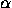# 10 Space Symmetries or Isometries

A transformation of space (invertible map of the plane to itself) that preserves distances is called an isometry of space. Every isometry of space is of one of the following types:

• the identity (which leaves every point fixed);
• a translation by a vector v;
• a rotation through an anglearound a line L;
• a screw motion through an anglearound a line L, with displacement d;
• a reflection in a line P;
• a glide-reflection in a line P with displacement vector v.
The identity is a particular case of a translation and of a rotation; rotations are particular cases of screw motions; and reflections are particular cases of glide-reflections. However, as in the plane case, is more intuitive to consider each case separately.

Next: 10.1 Formulas for Symmetries in Cartesian Coordinates
Up: Part II: Three-Dimensional Geometry
Previous: 9.5 Homogeneous Coordinates in SpaceThe Geometry Center Home Page

Silvio Levy
Wed Oct 4 16:41:25 PDT 1995

This document is excerpted from the 30th Edition of the CRC Standard Mathematical Tables and Formulas (CRC Press). Unauthorized duplication is forbidden.